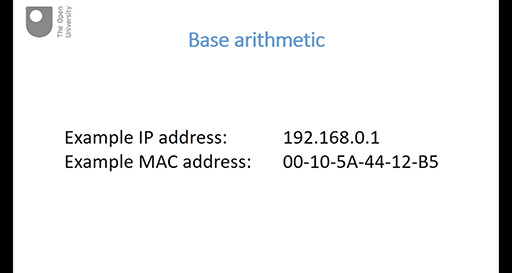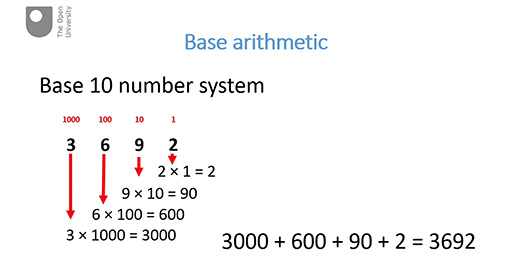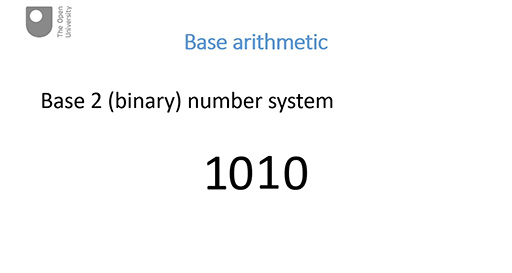Discovering computer networks: hands on in the Open Networking Lab

Start this free course now. Just create an account and sign in. Enrol and complete the course for a free statement of participation or digital badge if available.

Free course

# 7.1 Base arithmetic

In this part there is a slight side track from the focus on communications networks: instead you’ll take a short time to look at some arithmetic that will help you to understand the representation of network addresses. This is very fundamental arithmetic about how we count and how we represent numbers, and you may already be familiar with this. If this is the case, then you can treat this part as revision.

There are three videos in this part – each video is followed by an activity.

Watch the video below, which is about 3 minutes long.

## Base arithmeticSkip transcript

#### Transcript

Hello. In this video I’m going to introduce the idea of number systems and then go on to explain the base 10 number system.

These are examples of an IP address and a MAC address. You know from earlier sessions thtype="video" width="512"at they are just identification numbers but they’re represented in a way that you might not be familiar with. They use a different number base from the system we commonly use. By ‘number base’ I am referring to the number of unique symbols used to express numbers.

Most humans work with a base 10 number system – that’s a system that uses ten unique symbols: 0, 1, 2, 3, 4, 5, ,6 7, 8 and 9.The base 10 system is also known as the decimal or denary system. Through the use of just these ten symbols, we’re able to express infinitely large numbers. This is done by applying different weightings to the symbols depending on their position.

I’ll use the number three thousand six hundred and ninety two to illustrate this. The number in the rightmost position has the weighting of one. That is, any number in this position is multiplied by one. Here it is the number 2. 2 times 1 equals 2. So in our example, the symbol in this position (the 2) contributes 2 to the overall value of the group.

Moving leftwards, the number in the next position has the weighting of ten, so any number in this position is multiplied by 10. Here the number in this position is 9. (9 times 10 equals 90).So in our example, the symbol in this position contributes 90 to the overall value of the group.

The number in the next position to the left has a weighting of one hundred. So any number in this position is multiplied by one hundred. Here it’s 6. (6 times 100 equals 600) and therefore it contributes 600 to the overall total.

Finally, in this example, the number in the remaining position has a weighting of one thousand. Here the number is 3. So the number in this position is multiplied by one thousand (3 by 1000 equals 3000), thus contributing 3000 to the overall total.

Now we add together all the numbers derived from the successive weightings: 3000 + 600 + 90 + 2. This gives us three thousand six hundred and ninety two (3692).

Of course, we’re so used to expressing numbers in this way that we no longer need to go through this procedure in order to determine what the number is, but you may well have done this when you were first learning about arithmetic.

We call this form of arithmetic the base 10 number system, also commonly known as the ‘decimal’ or ‘denary’ number system.

End transcript

Interactive feature not available in single page view (see it in standard view).

## Activity 1 Test yourself

5 minutes

• In the base 10 (decimal) number 242, the symbol 2 represents different values depending on its position. What are these values?

• The symbol ‘2’ on the left is in third place so a weighting of 100 is applied to it, giving it a value of 200. The symbol ‘2’ on the right is in first place so a weighting of 1 is applied to it giving it a value of 2.

• Working in the base 10 (decimal) number system, in a six-symbol number such as 495,362, what weighting is applied to the leftmost symbol? What does the symbol ‘4’ represent in the number 495,362?

• The leftmost symbol in our example is in sixth place so a weighting of 100,000 is applied to this symbol. So in the decimal number 495,362, the ‘4’ represents 400,000.

Watch the video below, which is about 2 minutes long.

## Exponential notationSkip transcript

#### Transcript

Hello. This video introduces you to a system called ‘exponential notation’ which provides us with a useful shorthand for representing large numbers. I’ll start by revisiting the last part of the previous video.

Did you notice that as we moved from right to left the weighting applied was 10 times the previous one? This is what happens in a base 10 number system. We can express higher numbers simply by adding another position to the left with a weighting that is 10 times more than the previous position. But, as the weightings get larger, it becomes more and more difficult to show all the zeros. Did you notice how they were starting to get a bit squashed up in our example? And we were only using four places. It also becomes more difficult for us to interpret numbers when they’re followed by multiple zeros. They’re not easy for humans to count. Instead we use a convenient shorthand which I’ll explain.

In the decimal system, where we have a weighting of 1 which is the equivalent of no weighting at all, we use the shorthand 10 to the zero (ten to the power of zero). 10 to the zero is equal to 1. In fact any number raised to the power of zero is equal to 1. So, for example, 7 to the zero is equal to 1 and 12 to the zero is also equal to 1.

For a weighting of 10 we use the shorthand 10 to the one (ten to the power of one). 10 to the one equals 10 and raising it to the power of one has left it unchanged. In fact, any number raised to the power of one remains unchanged. That is 10 to the one = 10; and also, for example 7 to the one = 7; 12 to the one = 12.

For a weighting of 100 we use the shorthand 10 to the two. Ten to the power of two. That’s 10 times 10 which equals 100.

10 to the three equals 10 times 10 times 10 equals 1000 and so on.

This representation is known as exponential notation.

End transcript

Interactive feature not available in single page view (see it in standard view).

## Activity 2 Test yourself

5 minutes

• 8 × 103 =

• 8 × 103 = 8000

• 4 × 105 =

• 4 × 105 = 400,000

• 7 × 107 =

• 7 × 107 = 70,000,000

Watch the video below, which is about 3 minutes long.

## Base 2 arithmeticSkip transcript

#### Transcript

In this last video for this part I’m going to build on what you already know from the previous activities on base 10 arithmetic and exponential notation to explain base 2 arithmetic.

As you know, computers ‘think’ in zeros and ones. This means they can only use two symbols – a 0 and a 1 – to represent numbers. Number systems with only two symbols are known as base 2 or binary number systems. So how are we going to represent very large numbers using a binary system of only two symbols? I expect you’ve guessed or already know that we use a similar weighting system as we used with our base 10 system.

For example, let’s use the binary number 1010.

This time, as we move from right to left, the weighting is twice the value of the previous weighting. Remember it was 10 times the value in the decimal number system. This time I’m going to express the weightings using the exponential notation that you’ve just seen. The rightmost number has a weighting of 2 to the zero (that’s two to the power of zero). Remember I said previously that any number raised to the power of zero is one? So 2 to the zero is 1. There’s a 0 in this position so the value here is 0 times 1 equals 0. And the symbol in this position contributes 0 – nothing – to the overall value of the group.

The next number to the left has the weighting of 2 to the one. Remember I said previously that any number raised to the power of one remains unchanged? Therefore 2 to the one is 2. In this example there’s a 1 in this position so the value here is 1 times 2 equals 2, thus contributing 2 to the overall value of the group.

The next number to the left has the weighting of 2 to the two. That’s 2 times 2 equals 4. There’s a 0 in this position giving us 0 times 4 equals 0. So the symbol in this position contributes nothing to the total.

And finally, the next number to the left has the weighting of 2 to the three. That’s 2 times 2 times 2 equals 8. And here we have a 1 in this position contributing 1 times 8 equals 8 to the overall value.

Just as before, we add all the numbers derived from the successive weightings. This gives us 8 + 0 + 2 + 0 = 10, so the decimal equivalent of the binary number 1010 is 10.

Once you understand how the weighting system works in base arithmetic you can work in any number base.

In Part 3 of this session, you’ll meet the base 16 number system, known as hexadecimal.

End transcript

Interactive feature not available in single page view (see it in standard view).

## Activity 3 Test yourself

5 minutes

Give the denary equivalents of the following binary numbers:

• 1110

• 1110 = (1 × 23) + (1 × 22) + (1 × 21) + (0 × 20) = 8 + 4 + 2 + 0 = 14

• 1001

• 1001 = (1 × 23) + (0 × 22) + (0 × 21) + (1 × 20) = 8 + 0 + 0 + 1 = 9

• 101101

• This is more difficult as you need to apply some higher binary weightings of 25 (16) and 26 (32).

So 101101 = (1 × 25) + (0 × 24) + (1 × 23) + (1 × 22) + (0 × 21) + (1 × 20) = 32 + 0 + 8 + 4 + 0 + 1 = 45.

OPNL_1